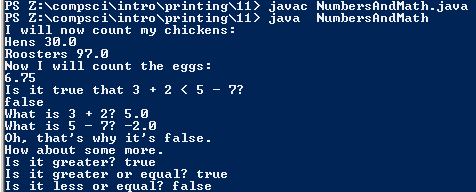# Assignemnt #11

## Code

```      /// name: Colin Hinton
/// period: 5
/// Project Name: NumbersAndMath
/// File Name: NumbersAndMath.java
/// Date Completed: 9/10/2015

public class NumbersAndMath
{
public static void main( String[] args )
{
//Printing Text
System.out.println( "I will now count my chickens:" );
//Printing Hens and the number computed
System.out.println( "Hens " + ( 25.0 + 30.0 / 6.0 ) );
//Printing Roosters, doing the 100 minius the remainder of 75/4
System.out.println( "Roosters " + ( 100.0 - 25.0 * 3.0 % 4.0 ) );
//Printing Text
System.out.println( "Now I will count the eggs:" );
//displaying a number computed
System.out.println( 3.0 + 2.0 + 1.0 - 5.0 + 4.0 % 2.0 - 1.0 / 4.0 + 6.0 );
// Print Text
System.out.println( "Is it true that 3 + 2 < 5 - 7?" );
//Stating if the value is greater than the other
System.out.println( 3.0 + 2.0 < 5.0 - 7.0 );
//Text, display number
System.out.println( "What is 3 + 2? " + ( 3.0 + 2.0 ) );
//Text, displaying other number
System.out.println( "What is 5 - 7? " + ( 5.0 - 7.0 ) );
//Text
System.out.println( "Oh, that's why it's false." );
// Text
System.out.println( "How about some more." );
//text and proof of greater than
System.out.println( "Is it greater? " + ( 5.0 > -2.0 ) );
//text and proof that it's greater than or equal
System.out.println( "Is it greater or equal? " + ( 5.0 >= -2.0 ) );
//text and proof that it's not less than or equal
System.out.println( "Is it less or equal? " + ( 5.0 <= -2.0 ) );
}
}
```

### Picture of the output